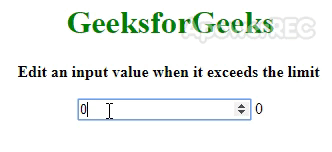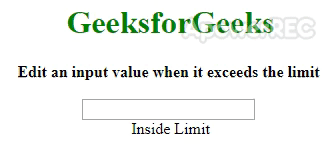# How to Reset an Input Value when Limit Exceeds using AngularJS ?

The task is to handle an input field if the number entered by the user in the input exceeds some limit using angularJS. We define a limitTo directive and use it on an HTML input element. This directive is used for all the handling of overflowing limits. The directive calls a function on the keypress event where we can check for the limits.

Example 1: This example sets the value of user input to 0 if its limit exceeds to 100.

 ` ` `<``html` `ng-app``=``"myApp"``> ` ` `  `<``head``> ` `    ``<``script` `src``= ` `"https://ajax.googleapis.com/ajax/libs/angularjs/1.7.9/angular.min.js"``> ` `    `` ` ` ` ` `  `<``body` `ng-controller``=``"MyController"``> ` `    ``<``center``> ` `        ``<``h1` `style``=``"color: green;"``> ` `            ``GeeksforGeeks ` `        `` ` `        ``<``b``> ` `            ``Edit an input value when ` `            ``it exceeds the limit ` `        `` ` `        ``<``br``><``br``> ` ` `  `        ``` `        ``<``input` `limit-to``=``"100"` `            ``type``=``"number"` `            ``ng-model``=``"val"``>  ` `        ``{{val}} ` `    `` ` `     `  `    ``<``script` `type``=``"text/javascript"``> ` `        ``angular.module('myApp', []) ` `            ``.controller('MyController',  ` `                ``['\$scope', function(\$scope) { ` `                         `  `                ``// Value to ng model the input ` `                ``\$scope.val; ` `            ``}]) ` `            ``.directive("limitTo", ['\$timeout', function(\$timeout) { ` `             `  `                ``// Declaration of custom directive ` `                ``return { ` `                    ``restrict: "A", ` `                    ``link: function(scope, elem, attrs) { ` `                        ``var limit = parseInt(attrs.limitTo); ` `                        ``elem.on("keypress", function(e) { ` `                            ``\$timeout(function() { ` `                                ``if (parseInt(e.target.value) > limit) ` `                                ``{ ` ` `  `                                    ``// Handle change here ` `                                    ``scope.val = 0; ` `                                    ``scope.\$apply(); ` `                                    ``e.preventDefault(); ` `                                ``} ` `                            ``}); ` `                        ``}); ` `                    ``} ` `                ``} ` `            ``}]); ` `    `` ` ` ` ` `  ` `

Output: As soon as the user exceeds 100, the value is set back to 0.Example 2: Here we change the text based on input limit. If the value of input is less than 0 then it goes to 0. If it’s value is greater than 100 then it goes to 100 otherwise it stays as it is.

 ` ` `<``html` `ng-app``=``"myApp"``> ` ` `  `<``head``> ` `    ``<``script` `src``= ` `"https://ajax.googleapis.com/ajax/libs/angularjs/1.7.9/angular.min.js"``> ` `    `` ` ` ` ` `  `<``body` `ng-controller``=``"MyController"``> ` `    ``<``center``> ` `        ``<``h1` `style``=``"color: green;"``> ` `          ``GeeksforGeeks ` `        `` ` `        ``<``b``> ` `          ``Edit an input value when it exceeds the limit ` `        `` ` `        ``<``br``><``br``> ` `         `  `        ``` `        ``<``input` `limit-to``=``"100"` `type``=``"number"` `ng-model``=``"val"``> ` `        ``<``br``> {{text}} ` `    `` ` `    ``<``script` `type``=``"text/javascript"``> ` `        ``angular.module('myApp', []) ` `            ``.controller('MyController', ['\$scope', function(\$scope) { ` `             `  `                ``// Value to ng model the input ` `                ``\$scope.val; ` `                ``\$scope.text = 'Inside Limit'; ` `            ``}]) ` `            ``.directive("limitTo", ['\$timeout', function(\$timeout) { ` `                 `  `                ``// Declaration of custom directive ` `                ``return { ` `                    ``restrict: "A", ` `                    ``link: function(scope, elem, attrs) { ` `                        ``var limit = parseInt(attrs.limitTo); ` `                        ``elem.on("keypress", function(e) { ` `                            ``\$timeout(function() { ` `                                ``if (parseInt(e.target.value) > limit) { ` `                                     `  `                                    ``// Handle change here if greater ` `                                    ``scope.text = "Outside limit (greater)"; ` `                                    ``scope.val = 100; ` `                                    ``scope.\$apply(); ` `                                    ``e.preventDefault(); ` `                                ``} else if (parseInt(e.target.value) < ``0``) { ` `                                    ``scope.text` `= ``"Outside limit (smaller)"``; ` `                                    ``scope.val` `= ``0` `                                    ``scope.\$apply(); ` `                                    ``e.preventDefault(); ` `                                ``} else { ` `                                    ``scope.text` `= ``"Inside Limit"``; ` `                                    ``scope.\$apply(); ` `                                    ``e.preventDefault(); ` `                                ``} ` `                            ``}); ` `                        ``}); ` `                    ``} ` `                ``} ` `            ``}]); ` `    `` ` ` ` ` `  ` `My Personal Notes arrow_drop_upCheck out this Author's contributed articles.

If you like GeeksforGeeks and would like to contribute, you can also write an article using contribute.geeksforgeeks.org or mail your article to contribute@geeksforgeeks.org. See your article appearing on the GeeksforGeeks main page and help other Geeks.

Please Improve this article if you find anything incorrect by clicking on the "Improve Article" button below.

Practice Tags :

Be the First to upvote.

Please write to us at contribute@geeksforgeeks.org to report any issue with the above content.